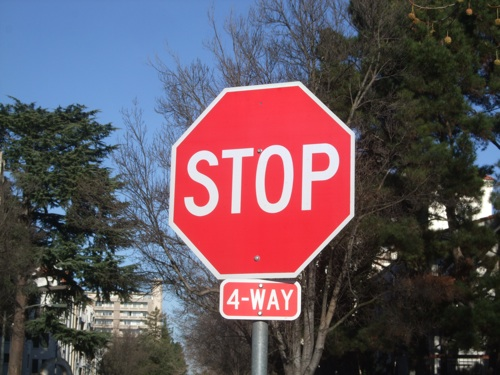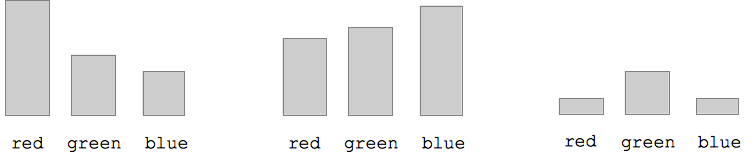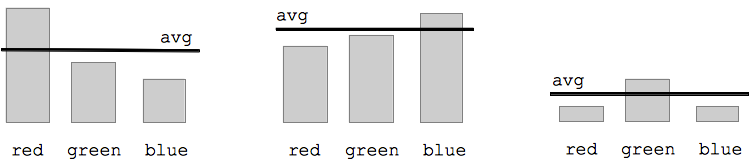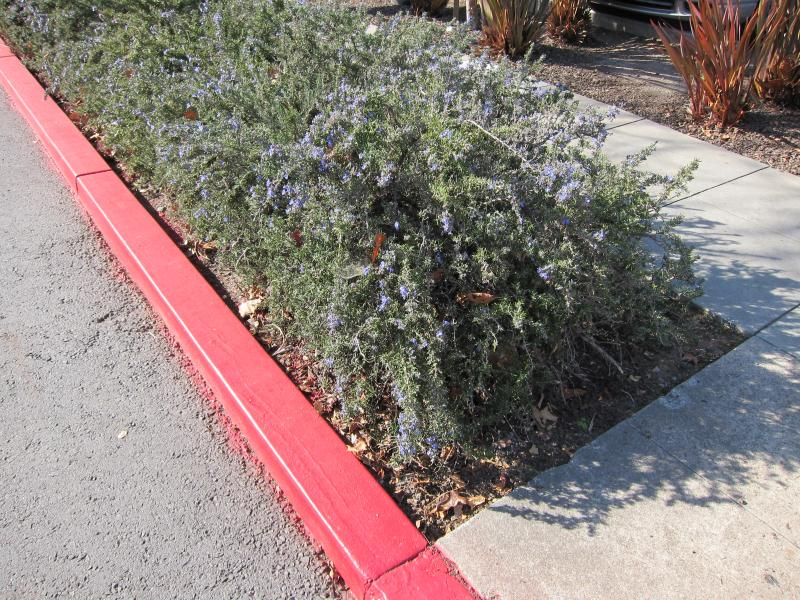# Image If-Statement Logic

• Theme 1: Bulk operation -- e.g. for-loop
•  Small body code, loop runs it over thousands of pixels
• Them 2: Logic test true/false -- e.g. if-statement (this section)
•  Run some code only if a test is true

Our use of loops thus far have allowed us to write a little bit of code which is run for many data points. That's one big theme in computer code. The if-statement, the topic of this section, will add a second theme: the ability to write a true/false test to control if a bit of code runs or not. Combined with the loop, the if-statement will greatly expand what we can do with code.

## If-Statement Syntax

The if-statement has a true/false test which controls if some code is run or not. Here is an example if-statement shown inside a for-loop:

```image = new SimpleImage("flowers.jpg");

for (pixel: image) {
if (pixel.getRed() > 160) {
pixel.setRed(0);
pixel.setGreen(0);
pixel.setBlue(0);
}
}

print(image);
```
• If-Statment syntax, in for-loop context
• `if`
• `(pixel.getRed() > 160)` -- "test" inside parenthesis
• Evaluate test -- true or false
• Tests just < (less than) or > (greater than) compare numbers (for now)
• `{ .. }` -- curly braces around then-code (indent)
• Run the then-code if the test is true (here sets to black)
• Syntax is all required (cs101 error message)
• This loop/if pattern solves many problems (select pixels)

For each pixel, the if-statement above retrieves the red value, and tests if the value is greater than 180. If the test is true, the if-statement runs the then-code inside the curly braces. If the test is false, the then-code is skipped. In this case, the then-code changes the pixel to be black.

## If Color Example

Here we'll use this picture of a stop sign:• Goal: change the red sign to be blue
• Test: `(pixel.getRed() > 160)`
• For each pixel, check if (red > 160)
• One strategy to test for red areas (needs improvement)
• Then-code: change the pixel to bright blue

 image = new SimpleImage("stop.jpg"); for (pixel: image) { if (pixel.getRed() > 160) { pixel.setRed(0); pixel.setGreen(0); pixel.setBlue(255); } } print(image);

• Above code works ok, but not perfectly
• The if-statement runs once for each pixel in the image.
• The test will be true or false for each pixel.
• Experiments:
• Nothing magic about 160 -- adjust it to get the result we want
• 1. Adjust the 160 value. If we change it to 220 or 250, will the if-test select more or fewer pixels than at 160? What if we set it to 100? 50?
• With a >, the 160 is like a hurdle we raise or lower
• When adjusting a test, consider if your goal is to make the test more or less restrictive
• Can also put any code we want for the then-code
• 2. Set red/green/blue all to 0

## The Problem With (red > 160) Test

Trying to change the stop sign to be blue, the test (red > 160) gets both white and red areas. The problem with that approach is that the red value is high in two kinds of cases -- the red part of the sign we want, but also parts of the scene that are near white. Recall that pure white is (255, 255, 255), so in whitish areas, all three values are high, so our (red > 160) test gets those too -- the white letters inside the sign are a clear example of this problem. Next we'll improve the test so it can distinguish the red and white parts of the sign.

## Color Bars

Suppose we have three pixels. Each pixel has the familiar red/green/blue values. Suppose these three values are graphed as bar graphs like this:Q: Each pixel has one of red/green/blue which is strongest. Look at the graphs to figure which shade dominates for each pixel. It's just whichever bar is tallest. Or to be specific, whichever bar is tallest relative to the others. The problem with our earlier strategy was that it just looked at absolute numbers -- (red > 160) -- failing to take into account this relative quality -- which bar is higher than the other two.

## Recall Average of Pixel Color

Recall this code which, inside a loop, computes the average of the red, blue, and green values and stores that number in a variable "avg".

```  avg = (pixel.getRed() + pixel.getGreen() + pixel.getBlue())/3;
```

## Color Bars With Average

Here are the three pixels again, but now the avg value is show as a line drawn across the bars.The avg gives us a handy way to tell if a color is high relative to the others. A pixel is reddish if the red value is over the avg. So here the first pixel is reddish and the other two are not. Or in terms of code, the test will look like `(pixel.getRed() > avg)` -- this will add the "relative to the other colors" quality that the previous test was missing.

## Color Avg Test Example - Stop

• Revisit the change sign to blue problem, using the avg
• Test: `(pixel.getRed() > avg * 1.1)`
• This works very well, picking out pixels with red cast
• ` * 1.1` adjustment factor, tighten/loosen to get look we want
• e.g. * 1.7 makes test more restrictive
• e.g. * 0.9 makes test less restrictive
• Here 1.4 looks pretty good

 image = new SimpleImage("stop.jpg"); for (pixel: image) { avg = (pixel.getRed() + pixel.getGreen() + pixel.getBlue())/3; if (pixel.getRed() > avg * 1.1) { pixel.setRed(0); pixel.setGreen(0); pixel.setBlue(255); } } print(image);

This is a better way to select the red parts of the sign. For each pixel, first compute the average value for that pixel. Then compare the red value to the average to decide if the pixel is reddish. Rather than checking the literal value of the red (e.g. 160), this checks if the red is relatively large compared to the other two colors .. does the pixel lean towards red. If the test is `(pixel.getRed() > avg)` we get all the areas that have every a tiny red cast, which is not restrictive enough. The fix is to multiply the avg by some factor to make it more restrictive, like this: `(pixel.getRed() > avg * 1.1)`. The specific value, 1.1, can be tuned by trying different values, until we get the look we want. Try the values: 0.9, 1.2, 1.4, 2, 2.5. The larger the value, the higher the bar is set to detect red pixels. By adjusting the * factor, we can zero in on the look we want. Here I think 1.4 looks pretty good.

## Color Avg Test Example - Curb

Suppose you are visiting Stanford and you park your car here, and get a parking ticket. Philosophically, they say that you are better off taking in events as they have actually happened. Nonetheless, here we'll try to fix history in code.Challenge: write code to detect the red curb, (a) change it to medium gray red=120 green=120 blue=120. (b) change just the red curb to be grayscale, which will look more realistic. Rather than changing the whole image to grayscale, we change just the red areas. Recall that the algorithm to change grayscale is to set red/green/blue values all to be the average value.

 image = new SimpleImage("curb.jpg"); for (pixel: image) { avg = (pixel.getRed() + pixel.getGreen() + pixel.getBlue())/3; if (pixel.getRed() > avg * 1.5) { pixel.setRed(120); pixel.setGreen(120); pixel.setBlue(120); } } print(image);

Solution code (b):

• Tune the * 1.5 to detect the red areas
• Setting to solid gray (120, 120, 120) looks a little crude
• Note that we put gray on the red plants to the right
• Our strategy chooses exclusively on pixel color, so necessarily get the red plants too
• (b) Rather than (120, 120, 120), change the red pixels to grayscale
• This looks much better, as it reflects the dark/light of the real curb
• Just draining out the red
• Conclusions:
• --`(pixel.getRed() > avg * 1.5)` pattern to detect pixel by color
• --The then-code can do anything we wish to the pixel

> exercises